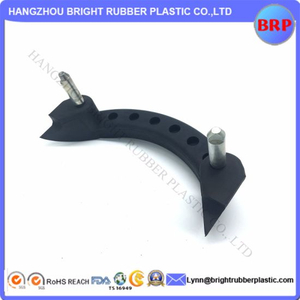## 产品分类# 定制金属粘合到橡胶零件上进行密封

• 根据客户要求
• > = 300％
• -40〜230摄氏度
• 欢迎光临
• BRP
• 标准出口包装，纸箱，托盘
• 根据客户要求
• 中国
• 4016931000

 材料 NR，NBR，SBR，EPDM，FKM，有机硅，HNBR，Viton等 尺寸 根据图纸 颜色 客制化 温度 公文：-40〜230摄氏度 特殊功能 /工作环境 无毒耐油性耐热性;抗磨性;耐老化 绝缘;耐酸碱密封;减震等 应用 用于家用，配件，管道及其他工业配件和汽车零件。 资质认证 SGS，RoHS和FDA。 交货时间 收到预付款后20-35天 起订量 100-1000片 包装明细 根据你的要求 装船 快递（DHL / UPS / FEDEX）/海运/空运 Port FOB上海或宁波

 E-优秀G-良好F-中等P-较差 材料特性 属性 丁腈橡胶 三元乙丙橡胶 CR 矽胶 FKM NR 丁苯橡胶 聚氨酯 丁腈橡胶 FVMQ IIR 阿夫拉斯 抗磨性 G G G P G E E E G P G G 耐酸性 F G F F E F F P E F G E 耐化学性 F E F G E F F F G E E E 耐寒性 G G F E P G G G G G G P 压缩永久变形 G G F G E G G F G G F G 动态特性 G G F P G E E E G P F G 电学特性 F G F E F G G F F E G E 伸长 G G G F F F F F G F G F 阻燃性 P P G F E P P P P G P E 耐热性 G G G E E F F F E E G E 抗渗性 G G G P G F F G G P E G 耐油性 E P F F E P P G E G P E 耐臭氧性 P E G E E P P E G E G E 抗撕裂性 F G F P F G G G F P G P 抗拉强度 G G G P G E E E E F G G 耐水性 G E F G F G G P E F G G 耐候性 F E E E E F F E G E G E

 材料 NR，NBR，SBR，EPDM，FKM，有机硅，HNBR，Viton等 尺寸 根据图纸 颜色 客制化 温度 公文：-40〜230摄氏度 特殊功能 /工作环境 无毒耐油性耐热性;抗磨性;耐老化 绝缘;耐酸碱密封;减震等 应用 用于家用，配件，管道及其他工业配件和汽车零件。 资质认证 SGS，RoHS和FDA。 交货时间 收到预付款后20-35天 起订量 100-1000片 包装明细 根据你的要求 装船 快递（DHL / UPS / FEDEX）/海运/空运 Port FOB上海或宁波

 E-优秀G-良好F-中等P-较差 材料特性 属性 丁腈橡胶 三元乙丙橡胶 CR 矽胶 FKM NR 丁苯橡胶 聚氨酯 丁腈橡胶 FVMQ IIR 阿夫拉斯 抗磨性 G G G P G E E E G P G G 耐酸性 F G F F E F F P E F G E 耐化学性 F E F G E F F F G E E E 耐寒性 G G F E P G G G G G G P 压缩永久变形 G G F G E G G F G G F G 动态特性 G G F P G E E E G P F G 电学特性 F G F E F G G F F E G E 伸长 G G G F F F F F G F G F 阻燃性 P P G F E P P P P G P E 耐热性 G G G E E F F F E E G E 抗渗性 G G G P G F F G G P E G 耐油性 E P F F E P P G E G P E 耐臭氧性 P E G E E P P E G E G E 抗撕裂性 F G F P F G G G F P G P 抗拉强度 G G G P G E E E E F G G 耐水性 G E F G F G G P E F G G 耐候性 F E E E E F F E G E G E

## 联系我们

  地址：中国杭州江干区金大塘3-1102
 电话：0571-88939871
 传真：0571-85267529
 Skype：Silvia.brightrubber
 邮件：silvia@brightrubberplastic.com

 QQ：529247255